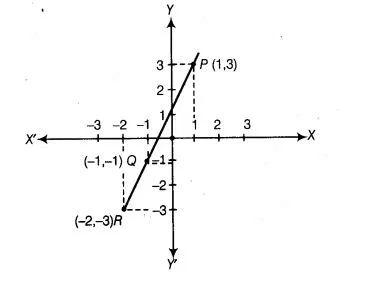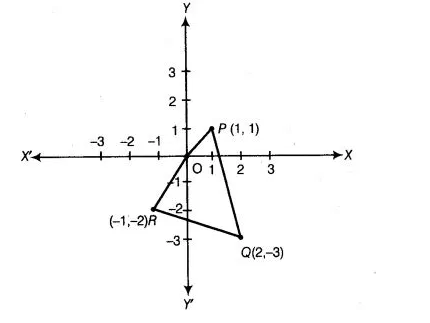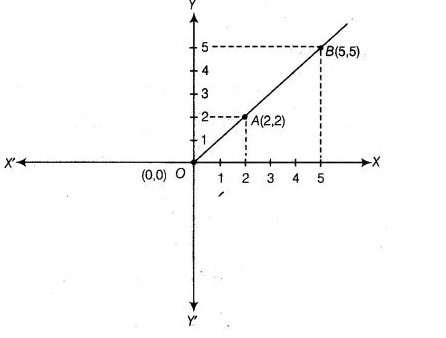# Plot the following points and check`
Question:

Plot the following points and check whether they are collinear or not

(i) (X 3), (-X -1), (-2, – 3)

(ii) (1,1), (2, – 3), (-X – 2)

(iii) (0,0),(2,2),(5,5)

Thinking Process

(i) Firstly, plot all three points on a graph paper and join them.

(ii) If it lives a straight line, then points are collinear otherwise non-collinear.

Solution:

(i) Plotting the points P (1, 3), Q (-1, -1) and R (-2, – 3) on the graph paper and join these points, we get a straight line. Hence, these points are collinear.(ii) Plotting the points P (1,1), 0 (2, – 3) and R (-1, -2) on the graph paper and join these three points, we get three lines i.e., the given points do not lie on the same line. So, given points are not collinear.(iii) Plotting the points 0 (0, 0), A (2,2)and 6 (5, 5)on the graph paper and join these points, we get a straight line. Hence, given points are collinear.